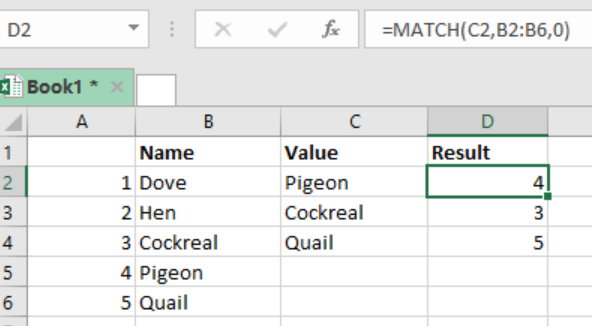Get instant live expert help with Excel or Google Sheets“My Excelchat expert helped me in less than 20 minutes, saving me what would have been 5 hours of work!”

#### Post your problem and you’ll get Expert help in seconds.

Your message must be at least 40 characters
Our professional Expert are available now. Your privacy is guaranteed.

# Excel MATCH Function

When we want to get the exact position of a given value in a column, row or table, we can use the Excel MATCH function. To fully understand how the function works, please read this post to the end.Figure 1: Using Excel MATCH function to find relative positions

## General syntax of the formula

`=MATCH (look_up, look_array, [match_type])`

Where;

• Lookup_value– value to match in look_up array
• Lookup_array– refers to the range of cells or array reference
• Match_type– shows how you want to match. Default is 1

## How the MATCH function works

We use the MATCH function when we want to find the relative position of an item in an array. This function is very flexible given that it has different matching modes.

When used together with INDEX, MATCH can also help to retrieve a value at the matched position.

## Different match types in MATCH function

• Match_type 1: this shows that the MATCH function will find the largest value which is less or equal to the lookup_value. Here, you will have to sort the lookup_array in ascending order.
• Match_type 0: here, the MATCH function will find the first value that is exactly equal to the lookup_value. You don’t have to sort the lookup_array when zero is your preferred match_type.
• Match_type -1: the MATCH function will find the smallest value which is greater than or equal to the lookup_value. You will have to sort the lookup_array in a descending order.

## Note

When the match_type is omitted, then it will be assumed to be 1.

Also, all these match_types will return the exact match of what you are seeking.

## Example

In this example, we want to find the relative positions of Dennis and Juliet. To do this, proceed as follows:

Step 1: Prepare your data sheet, complete with the names, value column as well as Result column

Step 2: Click in the cell where you want to get the result

Step 3: Write the formula in the active cell, i.e. `=MATCH (C2,A1:A5,0)`

Step 4: Press Enter

Step 5: Do the same for the other items.

Most of the time, the problem you will need to solve will be more complex than a simple application of a formula or function. If you want to save hours of research and frustration, try our live Excelchat service! Our Excel Experts are available 24/7 to answer any Excel question you may have. We guarantee a connection within 30 seconds and a customized solution within 20 minutes.

### Did this post not answer your question? Get a solution from connecting with the expert.Another blog reader asked this question today on Excelchat:
Solution examplesindex and match with duplicates. I need to use another column as a reference, so my return value has two match the value of two things for it to return
Solved by E. H. in 60 minsHello, I have a big spreadsheet in which I need to know how many patients came in each month based on provider. I am using the following formula but I still get the N/A error. =INDEX('No Show Appts Data'!D:E, MATCH(1, 'No Show Appts Data'!D:D='No Shows Data'!M2)*('No Shows Data'!E:E='No Shows Data'!N1),0)) No Show Appts Data is the name of the sheet where Column D is Month Year of date in question and Column E is the provider. No Shows Data is the sheet where I am making all the formula calculation where Column M is Month and Year and Column N is the provider in question and therefore M2 is the month in question and N1 the provider in question. How do I fix this error? Thanks
Solved by F. H. in 40 minsI need to find an INDEX function that will convert the Call Day (a number 1-7) to the actual weekday found in row 1 of the DayofWeek named range. I don't know if I'm supposed to use the MATCH function as well or not.
Solved by D. D. in 11 minsI have two columns with names and a third one with email addresses. For every name that matches, I need to copy the email address to an empty column next to the matching name. .
Solved by E. U. in 15 minshelp with INDEX(IndexArea,MATCH(\$M\$5,MatchRows,0),MATCH(\$M\$6,MatchColumns,0))
Solved by Z. Y. in 12 mins## Subscribe to Excelchat.coAnother blog reader asked this question today on Excelchat: